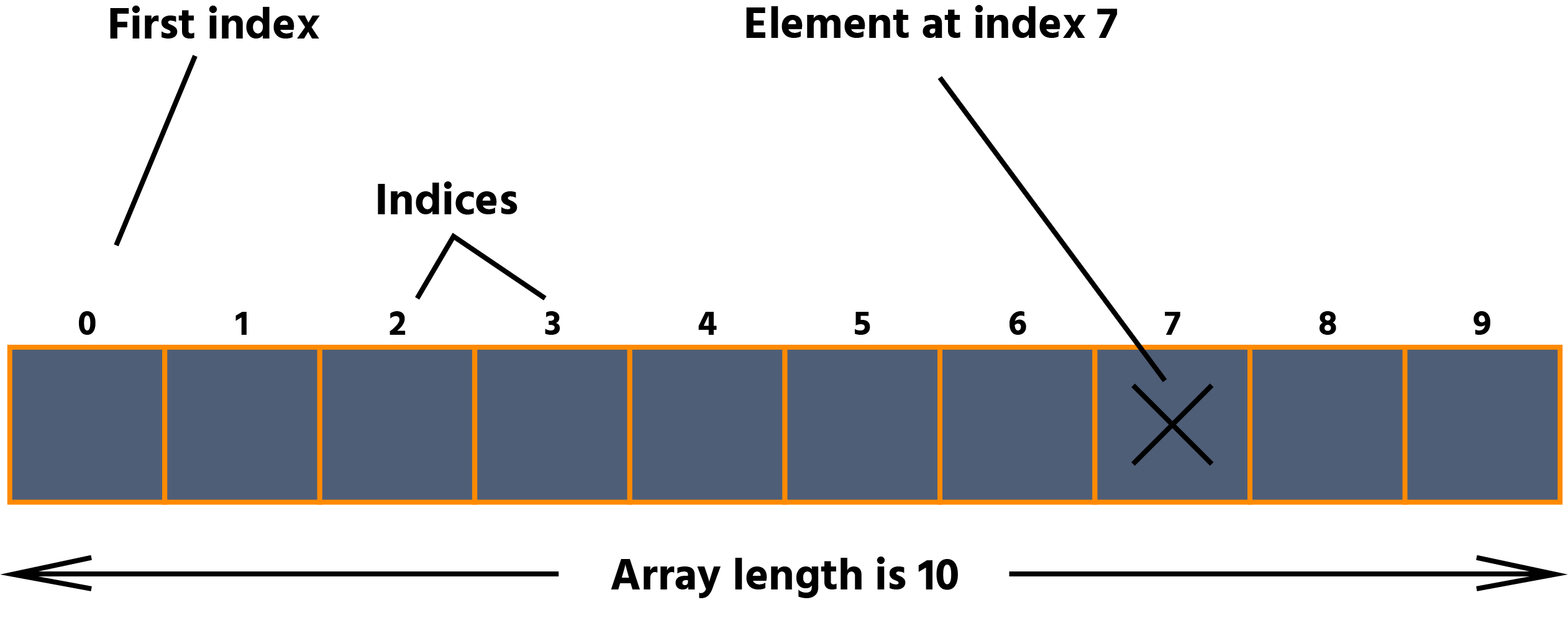Course Content

Introduction to JavaArrays 1/2

An array is a data structure that allows you to store a collection of elements of the same type, each of which is accessed by its numerical index.

It is used to store objects, and you can declare an array of any data type you want.

The position of an element in the array is called an index. The first element in the array is at index 0. The index of the last element in an array is always one less than the array's length.# Defining and creating arrays

You can declare arrays in two ways:

• `datatype[] arrayName`;
• `datatype arrayName[]`.Main.javaYou can create an array by using the `new` keyword, followed by the data type of the array and then the number of elements in square brackets.

The code for creating an array of integers:Main.javaThis creates an array object that can store 8 integer values. Array declaration and creation can be combined as the following code:Main.java## Literal array in Java

A literal array is an array that is declared and initialized in a single statement. It is created when the array is assigned a list of values. An array literal is a list of values enclosed in curly braces `{}`, and the values in the array literal are separated by commas `,`.Main.java## Access the elements in the array

The syntax of accessing elements in the array:

Note

Indeces start from 0. The first element is placed with the index 0, the second element is placed with the index 1, etc.

To calculate the index of an element, you can use the formula "N - 1" (where N is a number of an element).Main.javaAn array is a set of variables of a similar type. Each element is accessed using its index. An array index is a whole number that starts at zero. That means an array with five elements would have indices from 0 to 4.

For example:Main.java